#2017 iT 邦幫忙鐵人賽
DAY 26
7
Big Data

## 分類演算法:

• 決策樹、隨機森林
• 類神經網路
• 最近鄰居法
• 樸素貝氏分類及貝氏信念網路

## 決策樹(Decision Tree)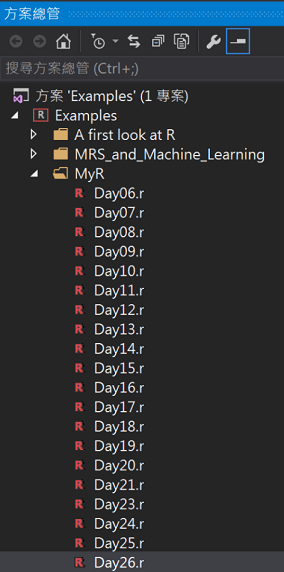## 使用決策樹演算法

``````#安裝package
install.packages("rpart")
install.packages("rpart.plot")
install.packages("rattle")

#載入library
library("rpart")
library("rpart.plot")
library("rattle")

#載入AER Package(AER: Applied Econometrics with R)
#install.packages("AER")
library(AER)

#(1)載入creditcard資料集(包含1,319筆觀察測試，共有12個變數)
data(CreditCard)

#假設我們只要以下欄位(card:是否核准卡片、信用貶弱報告數、年齡、收入(美金)、自有住宅狀況、往來年月)
bankcard <- subset(CreditCard, select = c(card, reports, age, income, owner, months))

#將是否核准卡片轉換為0/1數值
bankcard\$card <- ifelse(bankcard\$card == "yes", 1, 0);
``````

``````#(2)測試模型
#取得總筆數
n <- nrow(bankcard)
#設定隨機數種子
set.seed(1117)
#將數據順序重新排列
newbankcard <- bankcard[sample(n),]

#取出樣本數的idx
t_idx <- sample(seq_len(n), size = round(0.7 * n))

#訓練資料與測試資料比例: 70%建模，30%驗證
traindata <- newbankcard[t_idx,]
testdata <- newbankcard[ - t_idx,]
``````

``````#(3)建立決策樹模型
dtreeM <- rpart(formula = card ~ ., data = traindata, method = "class", control = rpart.control(cp = 0.001))

#(4)用rattle畫出厲害的決策樹(Rx: rxDTree)
fancyRpartPlot(dtreeM)
``````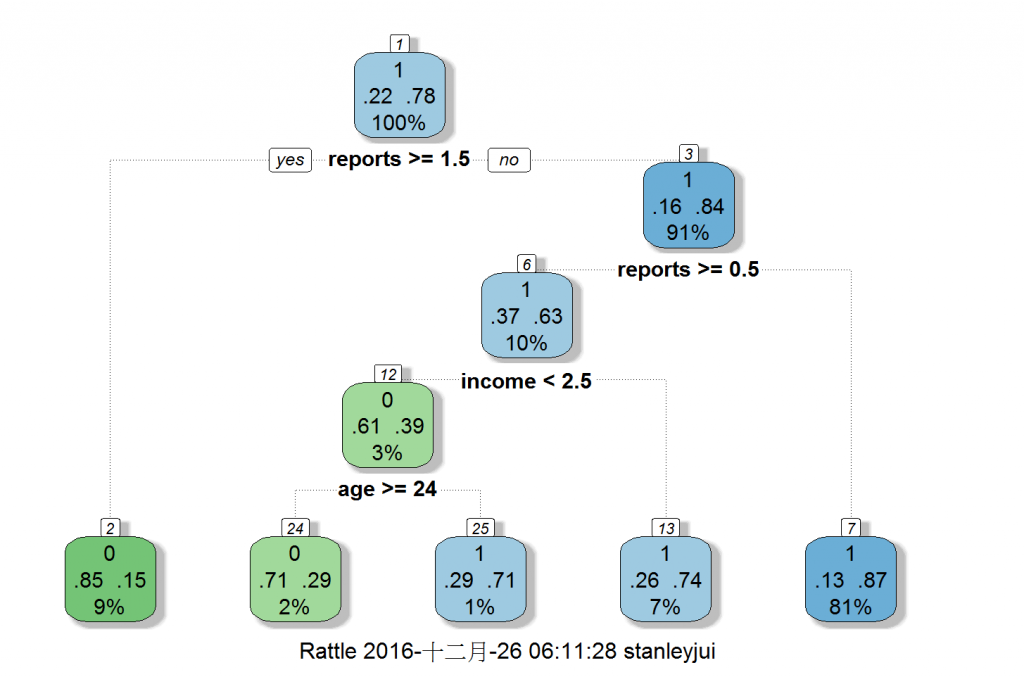## 決策樹預測及準確率

``````#(5)預測
result <- predict(dtreeM, newdata = testdata, type = "class")
#(6)建立混淆矩陣(confusion matrix)觀察模型表現
cm <- table(testdata\$card, result, dnn = c("實際", "預測"))
cm

#(6)正確率
#計算核準卡正確率
cm / sum(cm[, 2])

#計算拒補件正確率
cm / sum(cm[, 1])

#整體準確率(取出對角/總數)
accuracy <- sum(diag(cm)) / sum(cm)
accuracy

``````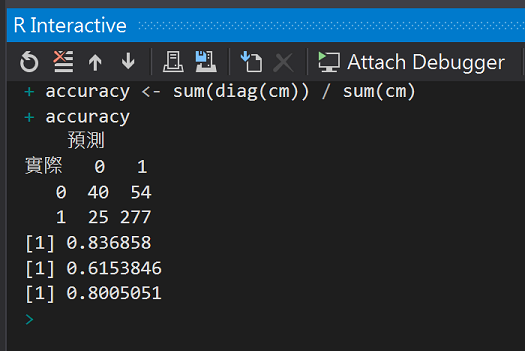## 條件推論樹(Conditional Inference Tree)

``````#install.packages("party")
library(party)

ct <- ctree(Species ~ ., data = iris)
plot(ct, main = "條件推論樹")
table(iris\$Species, predict(ct))

``````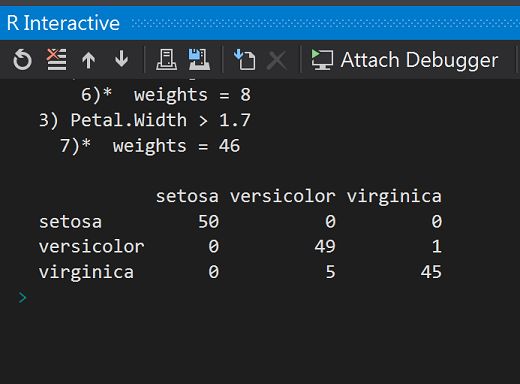## 隨機森林(Random Forests)

wiki

``````#載入隨機樹森林package
#install.packages("randomForest")
library(randomForest)
set.seed(1117)

#(2)跑隨機樹森林模型
randomforestM <- randomForest(card ~ ., data = traindata, importane = T, proximity = T, do.trace = 100)
randomforestM

#錯誤率: 利用OOB(Out Of Bag)運算出來的
plot(randomforestM)
``````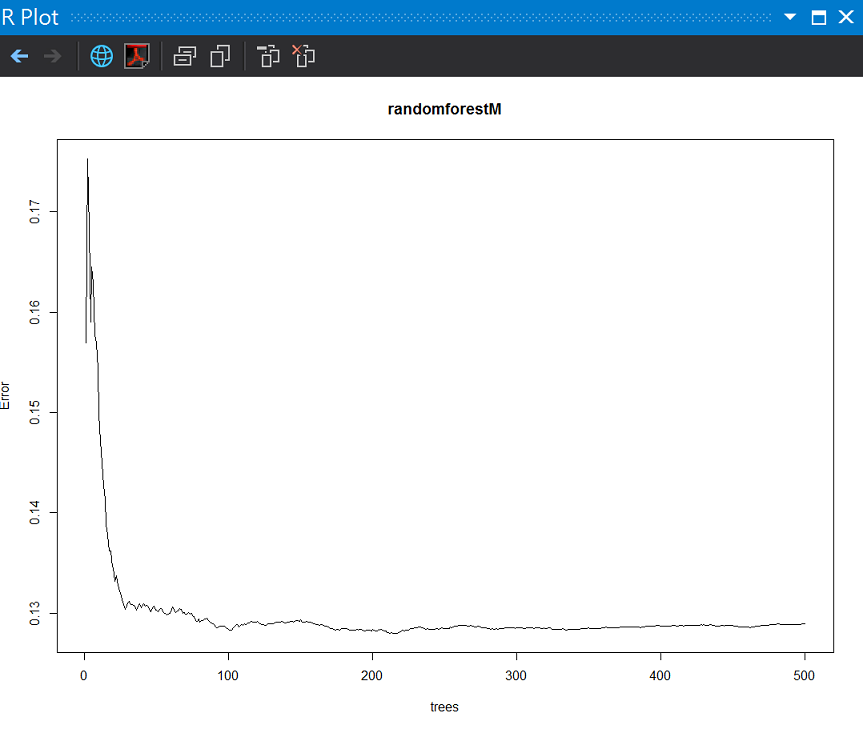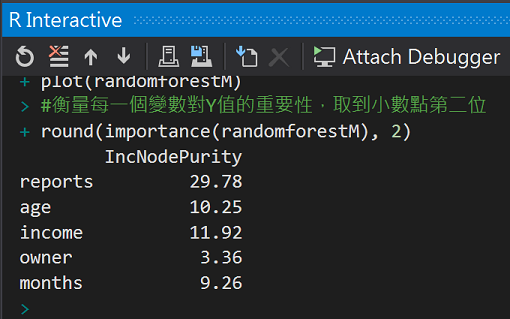``````#衡量每一個變數對Y值的重要性，取到小數點第二位
round(importance(randomforestM), 2)
````````````#(3)預測
result <- predict(randomforestM, newdata = testdata)
result_Approved <- ifelse(result > 0.6, 1, 0)

#(4)建立混淆矩陣(confusion matrix)觀察模型表現
cm <- table(testdata\$card, result_Approved, dnn = c("實際", "預測"))
cm

#(5)正確率
#計算核準卡正確率
cm / sum(cm[, 2])

#計算拒補件正確率
cm / sum(cm[, 1])

#整體準確率(取出對角/總數)
accuracy <- sum(diag(cm)) / sum(cm)
accuracy

``````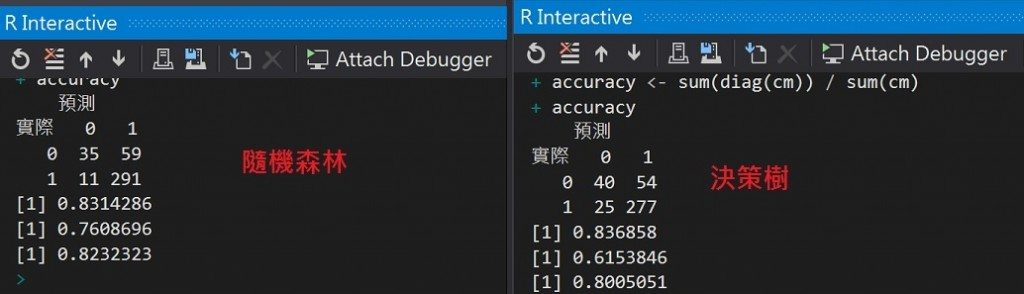## 整體學習(Ensemble learning)

[R 語言使用者的 Python 學習筆記 - 第 25 天] 機器學習（5）

• Bagging

### 參考:

CART Modeling